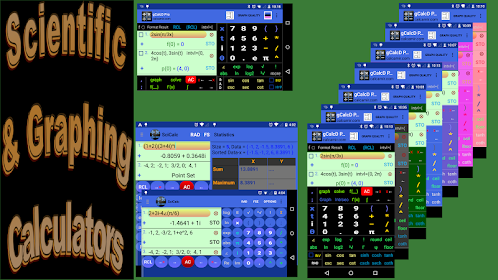# Graphing Calculator | Solve| DerivativesEveryone
50
The most easy-to-use, and the most powerful Graphing Calculator App for Android. In addition to functions, this Graphing Calculator is capable of graphing parametric equations and point sets using the Cartesian or polar coordinate systems.Furthermore, calculating x-intercepts, intersections, symbolic derivatives, definite integral, area, arc length, and curve fitting through a set of points, can all be easily performed by using this calculator as described below.

NOTE: To graph the expression in focus press the ‘graph’ button. To graph one or more selected expressions simultaneously, press the ‘Graph’ (capitalized) button.

This truly integrated Scientific/Graphing Calculator features graphing capabilities offered by no other graphing calculators and its advanced, user-friendly interface makes graphing and calculations as intuitive as possible.

• Calculate all the x-intercepts of the graph of a function on an interval with a single click.

• Calculate the points of intersection of the graphs of two functions.

• Calculate symbolic derivatives of functions and parametric equations and graph them.

• Calculate definite integral, area under (or enclosed by) the graphs of functions (or parametric curves) and arc length in both the Cartesian and polar cases.

• It is the only Graphing Calculator capable of finding and graphing the polynomial of the least degree passing through a set of points. In addition, you can use this Scientific Calculator to find and graph the best line (linear regression line) that fits a set of points according to Gauss' Least Squares Criteria.

Other Features

• This Scientific Calculator is also capable of calculating complex numbers in standard, polar or any other forms.

• The Calculator can display a table of values for functions and parametric equations even with complex expressions.

• The Calculator can display the result in scientific, engineering and fixed point notations.

• Calculate relevant expressions in degrees or radians mode.

• Apart from standard functions, this Graphing Calculator is equipped with Γ and Ψ functions.

•The Calculator also comes with an easy-to-use units converter (Time, Mass, Length, Velocity, and many more), and list of constants from various fields of sciences which can be used in your calculations.

3. Available functions

• Basic algebraic operations
• Power functions, x^r and E(x)
• Trigonometric functions and their inverses
• Hyperbolic functions and their inverses
• Exponential, e^x and exp(x), and logarithmic functions
• Factorial, the Gamma, Γ, and Psi, Ψ, functions
•Absolute value and step functions, round, floor, ceil

*********
Satisfaction Guaranteed – 100% Refund: If you are not satisfied with your purchase you can request a full refund by emailing the developer (just write "Refund", and state your purchase number and date of purchase) within a week from the time of the purchase. A full refund will be asked to be issued by Google Wallet once your email is received.
Collapse

Review Policy
4.3
50 total
5
4
3
2
1

## What's New

Discounted price : US\$0.99 - Regular price US\$3.99

• Bug fix; No more "wrong" error message when Scientific or Engineering notation selected for expressions with variables.
• Please rate this app and send feedback.
Collapse

Eligible for Family LibraryLearn More
Updated
January 9, 2017
Size
1.7M
Installs
10,000+
Current Version
v3.9.1.3
Requires Android
4.0.3 and up
Content Rating
Everyone
Permissions
Offered By
A.M.I.R
Developer
22 wood house rd London n12 0rgSee more